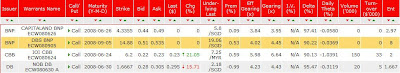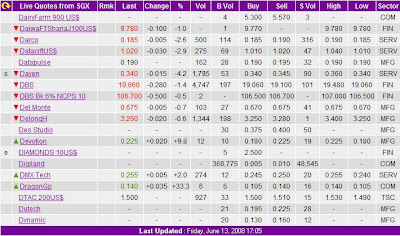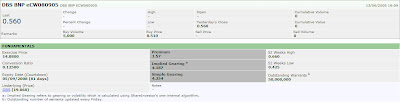## Sunday, 15 June 2008

### Actual Operation of Warrant Trading (Part 2)

This post is a continuation from where I stopped back in April. In this post, I will discuss about the bid/ask spread or in option trading world, it is commonly known as the slippage, of warrant trading.

Short term investors will find warrant trading attractive only if at least two conditions are satisfied. Firstly, there must be sufficient liquidity in the market so that warrants can easily change hands. Secondly, the bid/ask spreads must be narrow enough to keep transaction costs low. Since market making was introduced, liquidity is no longer a problem. Besides, as competition gets more and more intense, market makers are also maintaining their bid/ask spreads within a tight range. However, at times some warrants do trade with a rather wide spread. Well, then, how are bid/ask spreads determined?

The first factor to consider is delta. Given a conversion ratio of 1:1, the higher the delta (that is, close to 1 or 100%), the narrower the gap in the bid/ask spread between a warrant and its underlying. Let us say that the bid/ask spread of the underlying is S\$0.02. If the warrant has a very high delta, it’s bid/ask spread will be close to S\$0.02. However, if the conversion ratio is 10:1, the bid/ask spread of the warrant will be around S\$0.002.

Let use an actual example to see if that is the case. The screen capture below shows the bid/ask price of some warrant with delta close to 100% on June 13, 2008. The next screen capture shows the buy and sell price of some counters from SGX on the same date. Noticed in the first screen capture, three out of the four warrants in the screen capture that are very close to expiration have delta close to 100%. This should be the case since the three warrants are deep ITM with around two weeks to expiration. The only warrant with a delta close to 100% and more than two months to expiration is DBS BNP ECW080905. We shall use this as an example.Based on the second screen capture, the bid/ask spread of DBS is S\$19.10 – S\$19.06 = S\$0.04 and the entitlement ratio is 8. This means we should expect the bid/ask spread of the warrant to be around S\$0.04/8 = S\$0.005. However, based on the first screen capture, the bid/ask spread for DBS BNP ECW080905 is S\$0.535 - S\$0.51 = S\$0.025. This is 5 times more than what we expected. How about the theoretical bid/ask spread of a warrant? We can compute this by multiplying the delta of the warrant with the tick value of the underlying. Hence, the theoretical bid/ask spread for DBS BNP ECW080905 is 90.22% * S\$0.02 / 8 S\$0.002. Since the bid/ask spread of the warrant is also subject to the different tick values of different price ranges set by the stock exchange, we will expect the warrant bid/ask spread to be S\$0.005 (the minimum bid size for price range up to S\$0.99) which is consistent with the expected S\$0.005 bid/ask spread we calculated earlier on. Hence we need to find out why is the actual bid/ask spread 5 times more than the theoretical spread? This will lead us to the second factor.

The second factor to consider is the liquidity of the underlying. Once a warrant is issued, the issuer has to make the necessary hedging arrangements. Buying some holdings of the underlying is one of the methods. If the liquidity of the underlying is inadequate, the cost of hedging will be high. Hence, issuers will work out an estimate of the size of the float of the underlying they can buy or sell at the optimal price before setting the bid/ask spread of the warrant.

In the case of DBS BNP ECW080905, the delta is 90.22% and the bid/ask spread of the underlying (DBS) is S\$0.04; therefore the warrant bid/ask spread should be around S\$0.005. Since the conversion ratio is 8:1, this means for every 8 units of warrant the issuer needs 1 unit of DBS share to hedge its position. The outstanding warrant as on June 13, 2008 was 50, 000, 000. This is shown in the screen capture below.Assuming this value is accurate, this means that the accumulated overnight positions, held by investors rather than the issuer at the close of trading was 50 million units. This means if the issuer needs 50 000 000/8 = 6.25 million units of DBS shares to hedge its position since the listing of this warrant.

Let’s assume due to the insufficient liquidity in the market, the issuer can only buy 6 million units of DBS shares to hedge its position at optimal price says at its average price of around S\$18.80, computed based on the closing price of DBS since beginning of this year. This means the issuer has to pay an extra cost for the remaining 0.25 million units of DBS shares. If the issuer needs to pay the price of S\$19.00 to buy those units for hedging, the bid/ask spread of the warrant will widen to (19.00 – 18.80)/0.02 * 0.02 * 90.22% / 8 = S\$0.023 or about 5 ticks more.

The above are only assumptions made for the purpose of discussion and may not be necessary be true. Of course, sometimes an issuer may want to maintain a narrow spread for a particular warrant. So if the investors can spend a little time to observe how different issuers deal with the bid/ask spreads of their warrants, it would not be difficult to compare them and choose the most appropriate warrant to trade.